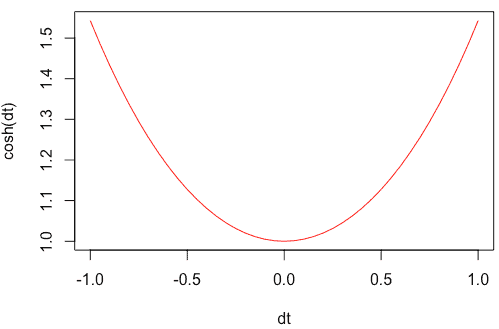# How to Use the cosh() Function in R

The cosh() function in R is “used to calculate the hyperbolic cosine of a number in radians. It accepts the numerical value as an argument and returns the hyper tangent of the cosine of that numeric value.

### Syntax

``cosh(x)``

### Parameters

x: It is a column to calculate.

### Return value

The cosh() function returns the hyperbolic cosine of a number (in radians) sent in as a parameter.

## Example 1: R program to use the cosh() function

Let’s calculate the cosh value of 1.

``cosh(1)``

Output

`` 1.543081``

Let’s calculate the cosh value of 0.

``cosh(0)``

Output

`` 1``

## Example 2: Using the cosh() function with a complex number

Define a complex value and pass that value to the cosh() function.

``````d <- 5 + 1i
cosh(d)``````

Output

`` 40.09581+62.43985i``

## Example 3: Using the cosh() function with a Vector

To create a Vector in R, you can use the c() function. Then pass that vector to the cosh() function.

``````rv <- c(-1, 0.5, 0, 0.5, 1)
cosh(rv)``````

Output

`` 1.543081 1.127626 1.000000 1.127626 1.543081``

## Example 4: Passing a pi to the cosh() function

The pi is an inbuilt constant in R programming, and its value is 3.141593.

Let’s find the pi constant’s cosh() value.

``cosh(pi)``

Output

`` 11.59195``

Let’s see another example of pi.

``cosh(pi / 4)``

Output

`` 1.324609``

## Plotting the cosh() function to a graph

We can use the seq() function to create a series of values and pass that to the plot() function, creating a line chart.

``````dt <- seq(-1, 1, by = 0.05)
plot(dt, cosh(dt), typ = "l", col = "red")
abline(v = 0, lty = 6, col = "blue")``````

OutputThat’s it.